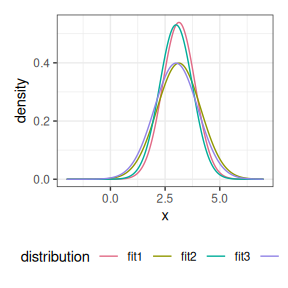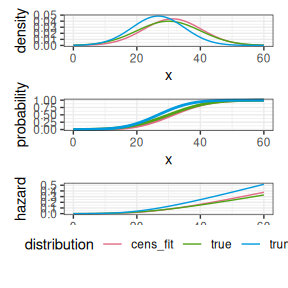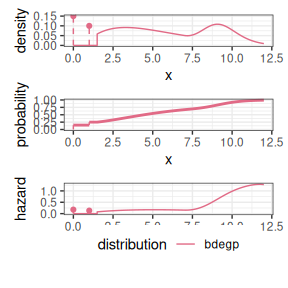# Working with Distributions

## Distributions

Distributions are a set of classes available in {reservr} to specify distribution families of random variables. A Distribution inherits from the R6 Class Distribution and provides all functionality necessary for working with a specific family.

A Distribution can be defined by calling one of the constructor functions, prefixed by dist_ in the package. All constructors accept parameters of the family as arguments. If these arguments are specified, the corresponding parameter is considered fixed in the sense that it need not be specified when computing something for the distribution and it will be assumed fixed when calling fit() on the distribution instance.

### Sample

For example, an unspecified normal distribution can be created by calling dist_normal() without arguments. This means the parameters mean and sd are considered placeholders. If we want to, e.g., sample from norm, we must specify these placeholders in the with_params argument:

library(reservr)
set.seed(1L)
# Instantiate an unspecified normal distribution
norm <- dist_normal()
x <- norm$sample(n = 10L, with_params = list(mean = 3, sd = 1)) set.seed(1L) norm2 <- dist_normal(sd = 1) x2 <- norm2$sample(n = 10L, with_params = list(mean = 3))

# the same RVs are drawn because the distribution parameters and the seed were the same
stopifnot(identical(x, x2))

### Density

The density() function computes the density of the distribution with respect to its natural measure. Use is_discrete_at() to check if a point has discrete mass or lebesgue density.

norm$density(x, with_params = list(mean = 3, sd = 1)) #>  0.3278626 0.3922715 0.2813724 0.1117603 0.3778620 0.2849269 0.3542572 #>  0.3037652 0.3380030 0.3807663 dnorm(x, mean = 3, sd = 1) #>  0.3278626 0.3922715 0.2813724 0.1117603 0.3778620 0.2849269 0.3542572 #>  0.3037652 0.3380030 0.3807663 norm$density(x, log = TRUE, with_params = list(mean = 3, sd = 1)) # log-density
#>   -1.1151607 -0.9358010 -1.2680761 -2.1913990 -0.9732262 -1.2555227
#>   -1.0377321 -1.1915002 -1.0847006 -0.9655696
norm$is_discrete_at(x, with_params = list(mean = 3, sd = 1)) #>  FALSE FALSE FALSE FALSE FALSE FALSE FALSE FALSE FALSE FALSE # A discrete distribution with mass only at point = x. dd <- dist_dirac(point = x) dd$density(x)
#>   1 0 0 0 0 0 0 0 0 0
dd$is_discrete_at(x) #>  TRUE FALSE FALSE FALSE FALSE FALSE FALSE FALSE FALSE FALSE diff_density() computes the gradient of the density with respect to each free parameter. Setting log = TRUE computes the gradient of the log-density, i.e., the gradient of log f(x, params) instead. norm$diff_density(x, with_params = list(mean = 3, sd = 1))
#> $mean #>  -0.20539076 0.07203805 -0.23512285 0.17828905 0.12450847 -0.23377349 #>  0.17267525 0.22427736 0.19461580 -0.11628160 #> #>$sd
#>   -0.19919475 -0.37904224 -0.08489705  0.17266080 -0.33683550 -0.09312311
#>   -0.27009027 -0.13817569 -0.22594681 -0.34525522

### Probability

With probability(), the c.d.f., survival function, and their logarithms can be computed. For discrete distributions, dist$probability(x, lower.tail = TRUE) returns $$P(X \le x)$$ and dist$probability(x, lower.tail = FALSE) returns $$P(X > x)$$.

norm$probability(x, with_params = list(mean = 3, sd = 1)) #>  0.2655087 0.5728534 0.2016819 0.9446753 0.6291140 0.2059746 0.6870228 #>  0.7698414 0.7176185 0.3800352 pnorm(x, mean = 3, sd = 1) #>  0.2655087 0.5728534 0.2016819 0.9446753 0.6291140 0.2059746 0.6870228 #>  0.7698414 0.7176185 0.3800352 dd$probability(x)
#>   1 1 0 1 1 0 1 1 1 1
dd$probability(x, lower.tail = FALSE, log.p = TRUE) #>  -Inf -Inf 0 -Inf -Inf 0 -Inf -Inf -Inf -Inf Gradients of the (log-)c.d.f. or survival function with respect to parameters can be computed using diff_probability(). norm$diff_probability(x, with_params = list(mean = 3, sd = 1))
#> $mean #>  -0.3278626 -0.3922715 -0.2813724 -0.1117603 -0.3778620 -0.2849269 #>  -0.3542572 -0.3037652 -0.3380030 -0.3807663 #> #>$sd
#>    0.20539076 -0.07203805  0.23512285 -0.17828905 -0.12450847  0.23377349
#>   -0.17267525 -0.22427736 -0.19461580  0.11628160

### Hazard

The hazard rate is defined by $$h(x, \theta) = f(x, \theta) / S(x, \theta)$$, i.e., the ratio of the density to the survival function.

norm$hazard(x, with_params = list(mean = 3, sd = 1)) #>  0.4463805 0.9183533 0.3524565 2.0200785 1.0188091 0.3588385 1.1318948 #>  1.3198083 1.1969728 0.6141740 norm$hazard(x, log = TRUE, with_params = list(mean = 3, sd = 1))
#>   -0.80658365 -0.08517306 -1.04282794  0.70313635  0.01863443 -1.02488292
#>    0.12389301  0.27748652  0.17979571 -0.48747702

### Fitting

The fit() generic is defined for Distributions and will perform maximum likelihood estimation. It accepts a weighted, censored and truncated sample of class trunc_obs, but can automatically convert uncensored, untruncated observations without weight into the proper trunc_obs object.

# Fit with mean, sd free
fit1 <- fit(norm, x)
# Fit with mean free
fit2 <- fit(norm2, x)
# Fit with sd free
fit3 <- fit(dist_normal(mean = 3), x)

# Fitted parameters
fit1$params #>$mean
#>  3.132203
#>
#> $sd #>  0.7405289 fit2$params
#> $mean #>  3.132203 fit3$params
#> $sd #>  0.752237 # log-Likelihoods can be computed on AIC(fit1$logLik)
#>  26.37096
AIC(fit2$logLik) #>  25.8626 AIC(fit3$logLik)
#>  24.68469

# Convergence checks
fit1$opt$message
#>  "NLOPT_SUCCESS: Generic success return value."
fit2$opt$message
#>  "NLOPT_SUCCESS: Generic success return value."
fit3$opt$message
#>  "NLOPT_SUCCESS: Generic success return value."

### Fitting censored data

You can also fit interval-censored data.

params <- list(mean = 30, sd = 10)
x <- norm$sample(100L, with_params = params) xl <- floor(x) xr <- ceiling(x) cens_fit <- fit(norm, trunc_obs(xmin = xl, xmax = xr)) print(cens_fit) #>$params
#> $params$mean
#>  31.25
#>
#> $params$sd
#>  9.112857
#>
#>
#> $opt #>$opt$par #> mean sd #> 31.250000 9.112857 #> #>$opt$value #>  362.9126 #> #>$opt$iter #>  5 #> #>$opt$convergence #>  1 #> #>$opt$message #>  "NLOPT_SUCCESS: Generic success return value." #> #> #>$logLik
#> 'log Lik.' -362.9126 (df=2)

### Fitting truncated data

It is possible to fit randomly truncated samples, i.e., samples where the truncation bound itself is also random and differs for each observed observation.

params <- list(mean = 30, sd = 10)
x <- norm$sample(100L, with_params = params) tl <- runif(length(x), min = 0, max = 20) tr <- runif(length(x), min = 0, max = 60) + tl # truncate_obs() also truncates observations. # if data is already truncated, use trunc_obs(x = ..., tmin = ..., tmax = ...) instead. trunc_fit <- fit(norm, truncate_obs(x, tl, tr)) print(trunc_fit) #>$params
#> $params$mean
#>  26.72871
#>
#> $params$sd
#>  8.242123
#>
#>
#> $opt #>$opt$par #> mean sd #> 26.728710 8.242123 #> #>$opt$value #>  203.8095 #> #>$opt$iter #>  9 #> #>$opt$convergence #>  1 #> #>$opt$message #>  "NLOPT_SUCCESS: Generic success return value." #> #> #>$logLik
#> 'log Lik.' -203.8095 (df=2)

attr(trunc_fit$logLik, "nobs") #>  62 ### Plotting Visualising different distributions, or parametrizations, e.g., fits, can be done with plot_distributions() # Plot fitted densities plot_distributions( true = norm, fit1 = norm, fit2 = norm2, fit3 = dist_normal(3), .x = seq(-2, 7, 0.01), with_params = list( true = list(mean = 3, sd = 1), fit1 = fit1$params,
fit2 = fit2$params, fit3 = fit3$params
),
plots = "density"
)# Plot fitted densities, c.d.f.s and hazard rates
plot_distributions(
true = norm,
cens_fit = norm,
trunc_fit = norm,
.x = seq(0, 60, length.out = 101L),
with_params = list(
true = list(mean = 30, sd = 10),
cens_fit = cens_fit$params, trunc_fit = trunc_fit$params
)
)# More complex distributions
plot_distributions(
bdegp = dist_bdegp(2, 3, 10, 3),
.x = c(seq(0, 12, length.out = 121), 1.5 - 1e-6),
with_params = list(
bdegp = list(
dists = list(
list(), list(), list(
dists = list(
list(
dist = list(
shapes = as.list(1:3),
scale = 2.0,
probs = list(0.2, 0.5, 0.3)
)
),
list(
sigmau = 0.4,
xi = 0.2
)
),
probs = list(0.7, 0.3)
)
),
probs = list(0.15, 0.1, 0.75)
)
)
)